﻿ Uncertainty vs Indeterminacy: A Journey from Fuzziness to NeutrosophyPublications are Open
Access in this journal
Article Versions
Export Article
• Normal Style
• MLA Style
• APA Style
• Chicago Style
Review Article
Open Access Peer-reviewed

### Uncertainty vs Indeterminacy: A Journey from Fuzziness to Neutrosophy

Michael Gr. Voskoglou
American Journal of Applied Mathematics and Statistics. 2022, 10(2), 65-68. DOI: 10.12691/ajams-10-2-4
Received July 19, 2022; Revised August 21, 2022; Accepted August 31, 2022

### Abstract

The present paper reviews the process that led from Zadeh’s fuzziness to Smarandache’s neutrosophy and discusses the future perspectives of the corresponding theories. Starting from the definitions of fuzzy set and of Atanassov’s intuitionistic fuzzy set, it proceeds to a detailed study of the concept of neutrosophic set, which takes in account the existing in real life indeterminacy. The basic operations of complement, union and intersection between neutrosophic sets are defined and the classical notion of topological space is extended to the notion of neutrosophic topological space. It is further shown that the fundamental concepts of convergence, continuity, compact topological space and Hausdorff topological space can be naturally extended to neutrosophic topological spaces.

### 1. Introduction

The existing in real life uncertainty is connected to the lack of knowledge and represents the total amount of potential information in a situation. This implies that a reduction of uncertainty due to a new evidence (e.g. receipt of a new message) indicates a gain of an equal amount of information.

The uncertain problems need imprecise methods that could deal with different types of uncertainties to increase the understanding of the outcomes. Probability theory was proved efficient for managing the cases of uncertainty due to randomness 1. Starting from the Zadeh’s fuzzy set (FS) 2 and fuzzy logic 3, however, several theories developed during the last 60 years for managing more effectively all the types of uncertainty; e.g. see 4.

FSs tackle successfully the uncertainty due to vagueness, which is created when one is unable to clearly differentiate between two classes, such as “a person of average height” and “a tall person”.

Atanassov introduced in 1986 the concept of intuitionistic FS (IFS) 5 by adding to Zadeh’s membership degree the degree of non-membership of each element of the universal set.

Smarandanche, motivated by the various neutral situations appearing in real life - like <friend, neutral, enemy>, <positive, zero, negative>, <small, medium, high>, <male, transgender, female>, <win, draw, defeat>, etc. - introduced in 1995 the concept of neutosophic set (NS) 6 by adding a third degree of indeterminacy between the degrees of membership and non-membership.

The present paper aims at reviewing the process that led from fuzziness to neutrosophy and at discussing the future perspectives of the corresponding theories. The rest of the paper is formulated as follows: Section 2 contains basic definitions and examples of FS and IFS. Section 3 is devoted to NSs and the definition of indeterminacy. Section 3 studies neutrosophic topological spaces (NTSs) and the article closes with the final conclusions and some hints for future research included in section 4.

### 2. Fuzzy and Intuitionistic Fuzzy Sets

The electrical engineer Lofti Aliasker Zadeh, Professor of Computer Science at the University of Berkeley, introduced in 1965 2 the concept of FS as follows:

Definition 1: Let U be the universal set of the discourse, then a FS Α in U is defined with the help of its membership function m: U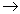[0,1] as the set of the ordered pairs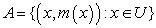(1)

The real number m(x) is called the membership degree of x in Α. The greater is m(x), the more x satisfies the characteristic property of Α. Many authors, for reasons of simplicity, identify a FS with its membership function.

A crisp subset A of U is a FS in U with membership function taking the values m(x)=1, if x belongs to A, and 0 otherwise. The classical operations on crisp sets (intersection, union, complement, etc.) are generalized in a natural way to FSs [ 7, Chapter 2].

The infinite-valued on the interval [0,1]. FL is defined with the help of the concept of FS. Through FL, the fuzzy terminology is translated by algorithmic procedures into numerical values, operations are performed upon those values and the outcomes are returned into natural language statements in a reliable manner 8. An important advantage of FL is that its rules are set in natural language with the help of linguistic, and therefore fuzzy, variables 9. For general facts on FSs and FL we refer to the book 7.

A difficulty appears, however, in FSs with the definition of the membership function, which is not unique, depending on the subjective perceptions of each person. In fact, the way of perceiving a concept (e.g. “tall”) is different from person to person, depending on the “signals” that each one receives from the real world about it. Thus, the only restriction about the definition of the membership function is to be compatible with the common logic; otherwise the corresponding FS does not provide a reliable representation of the corresponding real situation.

For a more accurate quantification of the uncertainty Kassimir Atanassov, Professor of Mathematics at the Bulgarian Academy of Sciences, introduced the concept of IFS as follows 5:

Definition 2: An IFS A in the universe U is defined with the help of its membership function m: U[0,1] and its non-membership function n: U[0,1] as the set of the ordered triples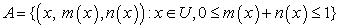(2)

One can write m(x) + n(x) + h(x) = 1, where h(x) is called the hesitation degree of x. When h(x) = 0, then the corresponding IFS is reduced to an ordinary FS. The characterization “intuitionistic” is due to the fact that an IFS contains the intuitionistic idea, as it incorporates the degree of hesitation.

For example, if A is the IFS of the clever students of a class and (x, 0.6, 0.2) ∈ A, then there is a 60% probability for the student x to be characterized as clever, a 20% probability to be characterized as not clever, and a 20% hesitation to be characterized as either clever or not. Most notions and operations concerning FSs can be extended to IFSs, which simulate successfully the existing imprecision in human thinking 10.

### 3. Neutrosophic Sets

3.1. Basic Concepts

The Romanian writer and mathematician Florentin Smarandache, Professor at the branch of Gallup of the New Mexico University, introduced the degree of indeterminacy/neutrality of the elements of the universe U and defined the concept of NS as follows 6:

Definition 3: A single valued NS (SVNS) A in U is of the form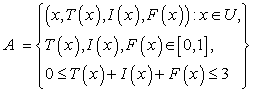(3)

In (3) T(x), I(x), F(x) are the degrees of truth, indeterminacy and falsity of x in A respectively, called the neutrosophic components of x. For simplicity, we symbolize the SVNS A by A<T, I, F>.

The etymology of the term “neutrosophy” comes from the adjective “neutral´ and the Greek word “sophia” (wisdom) and, according to Smarandanche who introduced it, means “the knowledge of neutral thought”.

For example, let U be the set of the players of a football team and let A be the SVNS of the good players of U. Then each player x of U is characterized by a neutrosophic triplet (t, i, f) with respect to A, with t, i, f in [0, 1]. For instance, x(0.6, 0.2, 0.4) ∈ A means that there is a 60% probability for x to be a good player, a 20% probability to be unknown if x is a good player and a 40% probability for x to be not a good player. In particular, x(0,1,0) ∈ A means that we do not know absolutely nothing about x’s affiliation with A.

Indeterminacy is defined to be in general everything which is between the two opposites of truth and falsity 11.

In an IFS indeterminacy is equal by default with hesitancy, i.e. we have I(x)=1- T(x) – F(x). Also, in a FS is I(x)=0 and F(x) = 1 – T(x), whereas in a crisp set is T(x)=1 (or 0) and F(x)= 0 (or 1). In other words, crisp sets, FSs and IFSs are special cases of SVNSs.

When the sum T(x) + I(x) + F(x) of the neutrosophic components of x ∈ U in a SVNS A on U is <1 then it leaves room for incomplete information about x, when is equal to 1 for complete information, and when is greater than 1 for parasconsistent (i.e. contradiction tolerant) information about x. A SVNS may contain simultaneously elements leaving room for all the previous types of information.

The difference between the general definition of a NS and the previously given definition of a SVNS is that in the general definition T(x), I(x) and F(x) may take values in the non-standard unit interval ]−0, 1+[ (including values <0 or >1) 6. This could happen in real world situations. For example, in a company with full-time work 40 hours per week for its employees, an employee, upon his work, could belong by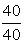=1 to the company (full-time job) or by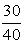<1 (part-time job) or by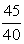>1 (over-time job). Assume further that a full-time employee caused a damage to his job’s equipment, the cost of which will be taken from his salary. Then, if the cost is equal to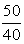of his weekly salary, the employee belongs this week to the company by -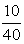<0.

NSs, apart from vagueness, manage as well the cases of uncertainty due to ambiguity and inconsistency. In ambiguity the existing information leads to several interpretations by different observers. For example, the statement “Boy no girl” written as “Boy, no girl” means boy, but written as “Boy no, girl” means girl. On the other hand, inconsistency appears when two or more pieces of information cannot be true at the same time. As a result the obtainable in this case information is conflicted or undetermined. For example, “the probability for raining tomorrow is 80%, but this does not mean that the probability for not raining is 20%, because they might be hidden weather factors”.

The difficulty, however, of defining properly the neutrosophic components of an object still exists, for the same reason as for the membership function of a FS mentioned in the previous section. The same also happens with IFSs, and generally for any generalization of FSs involving membership functions. At any case, theories for managing the uncertainty, related to FSs, have been developed where the definition of a membership function is not necessary: grey systems/numbers 13, or it is overpassed by using either a pair of sets which give the lower and the upper approximation of the original crisp set: rough sets 14, or a suitable set of linguistic parameters: soft sets 15. The present author has recently proposed a hybrid decision-making model using soft sets and grey numbers as tools 16.

3.2. Operations on Neutrosophic Sets

The classical operations on crisp sets are generalized to NSs 17. Here, for simplicity, we consider SVNSs and we define the subset and the complement of a SVNS, as well as the union and intersection of two SVNSs.

Definition 4: Let A<TA, IA, FA> and B<TB, IB, FB> be two SVNSs in the universe U. Then A is called a subset of B (A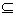B), if, and only if, TA(x)≤TB(x), IA(x)≤IB(x) and FA(x)≥FB(x), ∀ x ∈ U. If we have simultaneously AB and BA, then A and B are called equal SVNSs (A=B).

Definition 5: The complement of a SVNS A<TA, IA, FA> in U is the SVNS c(A)<FA, 1-IA, TA> in U.

Definition 6: Let A<TA, IA, FA> and B<TB, IB, FB> be two SVNSs in the universe U. Then the union A∪B is the SVNS C<TC, IC, FC> in U, with TC = max {TA, TB}, IC = max {IA, IB} and FC = min {FA, FB}.

Definition 7: Let A<TA, IA, FA> and B<TB, IB, FB> be two SVNSs in the universe U. Then the intersection A∩B is the SVNS C<TC, IC, FC> in U, with TC = min {TA, TB}, IC = min {IA, IB} and FC = max {FA, FB}.

It is straightforward to check that if A, B are crisp sets (FSs, IFSs) then the previous definitions are reduced to the corresponding definitions for crisp sets (FSs, IFSs).

Example 1: Let U= {x1, x2, x3} be the universal set and A={(x1, 0.3, 0.3, 0.6), ((x2, 0.5, 0.3, 0.4), (x3, 0.7, 0.2, 0.5)}, B={(x1, 0.6, 0.1, 0.2), (x2, 0.3, 0.2, 0.5), ((x3, 0.3, 0.1, 0.6)} be two SVNSs in U. Then:

i) Neither AB, nor BA (definition 4)

ii) c(A)={( x1, 0.6, 0.7, 0.3), (x2, 0.4, 0.7, 0.5), (x3, 0.5, 0.8, 0.7)} and c(B) ={(x1, 0.2, 0.9, 0.6), (x2, 0.5, 0.8, 0.3), (x3, 0.6, 0.9, 0.3)} (definition 5)

iii) A∪B={(x1, 0.6, 0.3, 0.6), (x2, 0.5, 0.3, 0.5), (x3, 0.3, 0.1, 0.5)} (definition 6)

iv) A∩B={(x1, 0.3, 0.1, 0.2), (x2, 0.3, 0.2, 0.4), (x3, 0.7, 0.2, 0.6)} (definition 7).

### 4. Neutrosophic Topological Spaces

FSs, FL and the related to them theories for managing the uncertainty have found many and important applications to almost all sectors of human activity (e.g. see 7, Chapter 6). Fuzzy mathematics, however, has also significantly developed in theoretical level giving important insights even to traditional branches of pure mathematics, like Algebra, Geometry, Analysis, Topology, etc.

Topological spaces is the most general category of mathematical spaces, in which fundamental mathematical concepts like convergence, continuity, compactness, etc. are defined 18. Metric spaces and manifolds are special forms of topological spaces satisfying some extra conditions.

It is recalled that the concept of a topological space is defined as follows:

Definition 8: A topology T on a non-empty set U is defined as a collection of subsets of U such that:

1. U and the empty set belong to T

2. The intersection of any two elements of T and the union of an arbitrary number of elements of T belong also to T.

Trivial examples are the discrete topology of all subsets of U and the non-discrete topology T= {U, ∅}. The usual topology on the set R of real numbers is defined as the set of all subsets A of R with the property that, for each a in A, there exists ε>0, such that (a-ε, a+ ε)A.

The elements of a topology T on U are called open subsets of U and their complements are called closed subsets of U. The pair (U, T) defines a topological space (TS) on U.

The concept of TS has been extended to fuzzy TS 19, to intuitionistic fuzzy TS 20, to soft TS 21, etc. Here we describe how one can extend the concept of TS to neutrosophic TS 22.

Definition 9: i) The empty NSN on the universe U is defined to be ∅N = {(x,0,0,1): x ∈ U}.

ii) The universal NS UN on U is defined to be UN = {(x,1,1,0): x ∈ U}.

It is straightforward to check that for each NS A in U is A∪UN = UN, A∩UN = A, A∪∅N = A and A∩∅N = ∅N.

Definition 10: A neutrosophic topology T on a non-empty set U is defined as a collection of NSs on U such that:

1. UN and ∅N belong to T, and

2. The intersection of any two elements of T and the union of an arbitrary number of elements of T belong also to T.

Trivial examples are the discrete neutrosophic topology of all NSs in U and the non-discrete neutrosophic topology T= {UN, ∅N}.

The elements of a neutrosophic topology T on U are called open NSs in U and their complements are called closed NSs in U. The pair (U, T) defines a neutrosophic topological space (NTS) on U.

Example 2: Let U = {u} and let A = {(u ,0.5,0.5,0.4)},

B = {(u ,0.4,0.6,0.8)}, C = {(u ,0.5,0.6,0.4)},

D ={(u,0.4,0.5,0.8)} be NSs in U. Then it is straightforward to check that the collection T = {∅N, UN, A, B, C, D} is a neutrosophic topology on U.

We close by extending the concepts of convergence, continuity, compact TS and Hausdorff TS to NTSs.

Definition 11: Given two NSs A and B of the NTS (U, T), B is said to be a neighborhood of A, if there exists an open NS O such that AO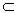B. Further, we say that a sequence {An} of NSs of (U, T) converges to the NS A of (U, T), if there exists a positive integer m such that for each integer n≥m and each neighborhood B of A we have that AnB.

The following theorem generalizes the Zadeh’s extension principle for FSs 7 to NSs:

Theorem 1: Let U and V be two non-empty crisp sets and let g: UV be a function. Then g can be extended to a function G mapping NSs in U to NSs sets in V.

Proof: Let A<TA, IA, FA> be a NS in U. Then its image G(A) is a NS B in V, whose neutrosophic components are defined as follows: Given v in V, consider the set g-1(v)={u ∈ U: g(u)=v}. If g-1(v)=∅, then TB(v)=0, and if g-1(v)≠∅, then TB(v) is equal to the maximal value of all TA(u) such that u ∈ g-1(v). Conversely, the inverse image G-1(B) is the NS A in U with truth membership function TA(u)=TB(g(u)), for each u ∈ U. In an analogous way one can determine the neutrosophic components IB and FB of B.

Definition 12: Let (U,T) and (V,S) be two NTSs and let g be a function g: UV. According to Theorem 1, g can be extended to a function G which maps NSs of U to NSs of V. We say then that g is a neutrosophicaly-continuous function, if, and only if, the inverse image of each open NS of V through G is an open NS of U.

Definition 13: A family A = {Ai, i∈I} of NSs of a NTS (U, T) is called a cover of U, if U =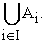If the elements of A are open NSs, then A is called an open cover of U. Also, each NS subset of A which is also a cover of U is called a sub-cover of A. The NTS (U, T) is said to be compact, if every open cover of U contains a sub-cover with finitely many elements.

Definition 14: A NTS (U, T) is called a T1-NTS, if, and only if, for each pair of elements u1, u2 of U, u1≠u2, there exist at least two open NSs O1 and O2 such that u1∈O1, u2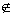O1 and u2∈O2, u1O2.

Definition 15: A NTS (U, T) is called a T2-NTS, if, and only if, for each pair of elements u1, u2 of U, u1≠ u2, there exist at least two open NSs O1 and O2 such that u1∈O1, u2∈O2 and O1∩O2 = ∅N.

A T2-NTS is also called a Hausdorff or a separable NTS. Obviously a T2-NTS is always a T1-NTS.

### 5. Discussion and Conclusions

In this work the concept of NS in the universe U, introduced by Smarandanche in 1995, which describes the existing in real life indeterminacy, was studied. The basic operations between NSs were presented and the classical notion of TS was extended to NTS. It was further shown that convergence, continuity, compact TS and Hausdorff TS can be naturally extended to NTSs.

It looks in general that the combination of two or more of the alternative theories developed for tackling the existing in real life uncertainty, is a promising tool for obtaining better results in a variety of human activities characterized by uncertainty (e.g. see 16). Consequently this could be a fruitful area for future research.

### References

  Kosko, B., Fuzziness Vs Probability, Int. J. of General Systems, 17(2-3), 211-240, 1990. In article View Article  Zadeh, L.A., Fuzzy Sets, Information and Control, 8, 338-353, 1965 In article View Article  Zadeh, L.A., Outline of a new approach to the analysis of complex systems and decision processes, IEEE Trans. Syst. Man Cybern., 3, 28-44, 1973. In article View Article  Voskoglou, M.Gr., Generalizations of Fuzzy Sets and Related Theories, in M. Voskoglou (Ed.), An Essential Guide to Fuzzy Systems, 345-352, Nova Publishers, N.Y., 2019. In article  Atanassov, K.T., Intuitionistic Fuzzy Sets, Fuzzy Sets and Systems, 20(1), 87-96, 1986. In article View Article  Smarandache, F., Neutrosophy/Neutrosophic probability, set, and logic, Amer. Res. Press, Rehoboth, USA, 1998. In article  Klir, G. J. & Folger, T. A., Fuzzy Sets, Uncertainty and Information, Prentice-Hall, London, 1988. In article  Kosko, B., Fuzzy Thinking: The New Science of Fuzzy Logic, Hyperion: New York, 1993. In article View Article  Zadeh, L.A., The Concept of a Linguistic Variable and itsApplication to Approximate Reasoning, Information Science, 8, 199-249, 1975. In article View Article  Atanassov, K.T., Intuitionistic Fuzzy Sets, Physica-Verlag, Heidelberg, , N.Y, 1999. In article View Article  Smarandache, F., Indeterminacy in Neutrosophic Theories and their Applications, International Journal of Neutrosophic Science, 15(2), 89-97, 2021. In article View Article  Cuong, B., Picture Fuzzy Sets, J. of Computer Science and Cybernetics, 20(4), 409-420, 2014. In article View Article  Deng, J., Control Problems of Grey Systems, Systems and Control Letters, 288-294, 1982. In article View Article  Pawlak, Z., Rough Sets: Aspects of Reasoning about Data, Kluer Academic Publishers, Dordrecht, 1991. In article View Article  Molodtsov, D., Soft Set Theory-First Results, Computers and Mathematics with Applications, 37(4-5), 19-31, 1999. In article View Article  Voskoglou, M.Gr., A Combined Use of Soft Sets and Grey Numbers in Decision Making, Journal of Computational and Cognitive Engineering, 2022. In article  Wang, H., Smarandanche, F., Zhang, Y., Sunderraman, R., Single Valued Neutrosophic Sets, Review of the Air Force Academy (Brasov), 1(16), 10-14, 2010. In article  Willard, S., General Topology, Dover Publ. Inc., N.Y., 2004. In article  Chang, S.L., Fuzzy Topological Spaces, Journal of Mathematical Analysis and Applications, 24(1), 182-190, 1968. In article View Article  Luplanlez, F.G, On intuitionistic fuzzy topological spaces, Kybernetes, 35(5), 743-747, 2006. In article View Article  Shabir, M. & Naz M., On Soft Topological Spaces, Computers and Mathematics with Applications, 61, 1786-1799, 2011. In article View Article  Salama, A.A., Alblowi, S.A., Neutrosophic Sets and Neutrosophic Topological Spaces, IOSR Journal of Mathematics, 3(4), 31-35, 2013. In article View ArticleThis work is licensed under a Creative Commons Attribution 4.0 International License. To view a copy of this license, visit http://creativecommons.org/licenses/by/4.0/